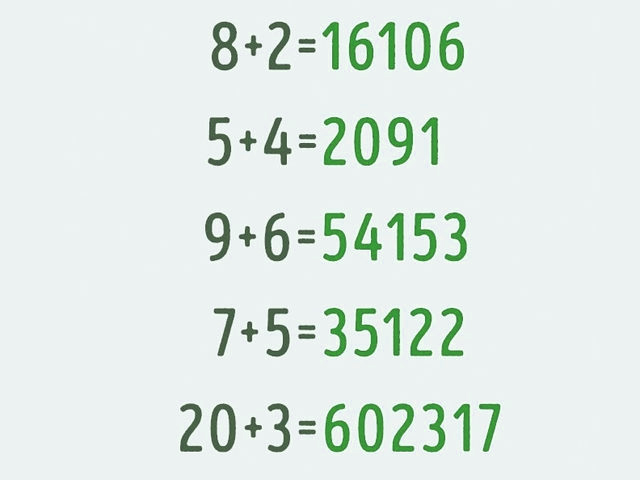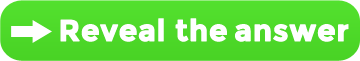# Only A Real Genius Can Solve This Math Equation

Try to find the connection between these equations:Hunt 1:
Try not to focus on the big number in the “answer”. It makes more sense not to think of it as one big number but several smaller ones put together.

Hunt 2:
It’s actually quite simple: every number after the equals sign actually represents the results of three sums formed by multiplying, adding, and subtracting the two number before the equals sign.

Click to reveal the solution: